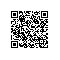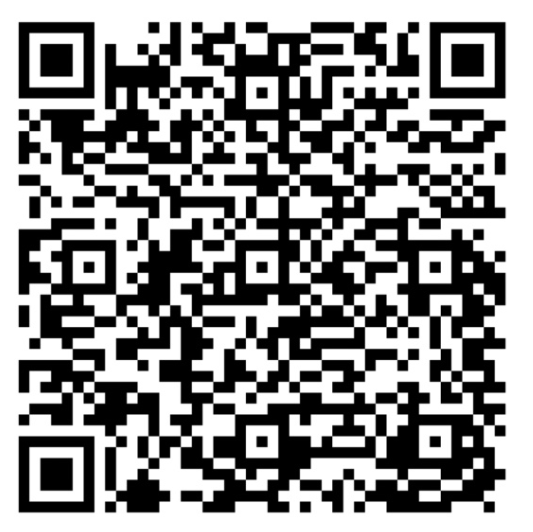# 如何判断网页是否已经滚动到浏览器底部了• $(window).height(); //用于获取浏览器显示区域的高度 •$(window).width(); //用于获取浏览器显示区域的宽度
• $(document.body).height(); //获取页面文档的高度 •$(document.body).width(); //获取页面文档的宽度
• $(document).scrollTop(); //获取垂直滚动条到顶部的垂直距离 •$(document).scrollLeft(); //获取水平滚动条到左边的水平距离

1. $(window).scroll(function(){ 2. var h=$(document.body).height();//网页文档的高度
3.                 var c = $(document).scrollTop();//滚动条距离网页顶部的高度 4. var wh =$(window).height(); //页面可视化区域高度
5.
6.                 if (Math.ceil(wh+c)>=h){
8.                 }
9.             })

1. $(window).scroll(function(){ 2. var h=$("#div").offset().top;//id为div的元素距离网页顶部的距离
3.                 var c = $(document).scrollTop();//滚动条距离网页顶部的高度 4. var wh =$(window).height(); //页面可视化区域高度
5.
6.                 if (Math.ceil(wh+c)>=h){
8.                 }
9.             })

1. (function ($) { 2. //backcall是回调函数，count表示回调函数被执行的次数，count只有元素在屏幕范围之外的时候才起作用 3.$.fn.inBottom = function (backcall){
4.         //判断当前元素是否在目前屏幕可视化区域之内
5.         if(this.offset().top >= $(window).height()){ 6. this.inScroll(backcall,count); 7. }else 8. this.inWindow(backcall); 9. } 10. }; 11. //直接加载回调函数 12.$.fn.inWindow = function (backcall){
13.         backcall();
14.
15.     };
16.     //滚动监听滑动条，元素滚动到屏幕底部的时候，加载回调函数
17.     $.fn.inScroll = function (backcall,count) { 18. var$this=this;
19.         $(window).scroll(function(){ 20. //获得这个元素到文档顶部的距离 21. var awayDocTop=$this.offset().top
22.         //获得滚动条的top
23.         var sTop=$(document).scrollTop(); 24. //获得可视化窗口的高度 25. var wh=$(window).height();
26.         if(Math.ceil(wh+sTop)>=awayDocTop){
27.             if(count>0){
28.                 backcall();
29.                 count--;
30.             }
31.         };
32.     });
33.     };
34. })(jQuery);

1. \$("#div").inBottom(function(){
3. },1);使用钉钉扫一扫加入圈子
+ 订阅